Have a question about one of the blog posts? Chat here with me.

# How to basic: bar plots

R

This blog post shows how to make bar plots and area charts. It’s mostly a list of recipes, indented for myself. These are plots I have often to do in reports and would like to have the code handy somewhere. Maybe this will be helpful to some of you as well. Actually, this post is exactly how I started my blog post. I wanted to have a repository of recipes, and with time the blog grew to what it is now (tutorials and me exploring methods and datasets with R).

## Bar charts

Bar charts are quite simple plots, but there are enough variations of them that they deserve one single blog post. However, don’t expect many explanations.

Let’s first start by loading some data, and the usually required packages:

``````library(tidyverse)
library(lubridate)
library(janitor)
library(colorspace)``````
``data(gss_cat)``

Very often, what one wants to show are counts:

``````gss_cat %>%
count(marital, race)``````
``````## # A tibble: 18 x 3
##    marital       race      n
##  * <fct>         <fct> <int>
##  1 No answer     Other     2
##  2 No answer     Black     2
##  3 No answer     White    13
##  4 Never married Other   633
##  5 Never married Black  1305
##  6 Never married White  3478
##  7 Separated     Other   110
##  8 Separated     Black   196
##  9 Separated     White   437
## 10 Divorced      Other   212
## 11 Divorced      Black   495
## 12 Divorced      White  2676
## 13 Widowed       Other    70
## 14 Widowed       Black   262
## 15 Widowed       White  1475
## 16 Married       Other   932
## 17 Married       Black   869
## 18 Married       White  8316``````

Let’s lump marital statuses that appear less than 10% of the time into an “Other” category:

``````(
counts_marital_race <- gss_cat %>%
mutate(marital = fct_lump(marital, prop = 0.1)) %>%
count(marital, race)
)``````
``````## # A tibble: 12 x 3
##    marital       race      n
##  * <fct>         <fct> <int>
##  1 Never married Other   633
##  2 Never married Black  1305
##  3 Never married White  3478
##  4 Divorced      Other   212
##  5 Divorced      Black   495
##  6 Divorced      White  2676
##  7 Married       Other   932
##  8 Married       Black   869
##  9 Married       White  8316
## 10 Other         Other   182
## 11 Other         Black   460
## 12 Other         White  1925``````

The simplest bar plot:

``````ggplot(counts_marital_race) +
geom_col(aes(x = marital, y = n, fill = race)) +
scale_fill_discrete_qualitative(palette = "Dark 3") +
brotools::theme_blog()``````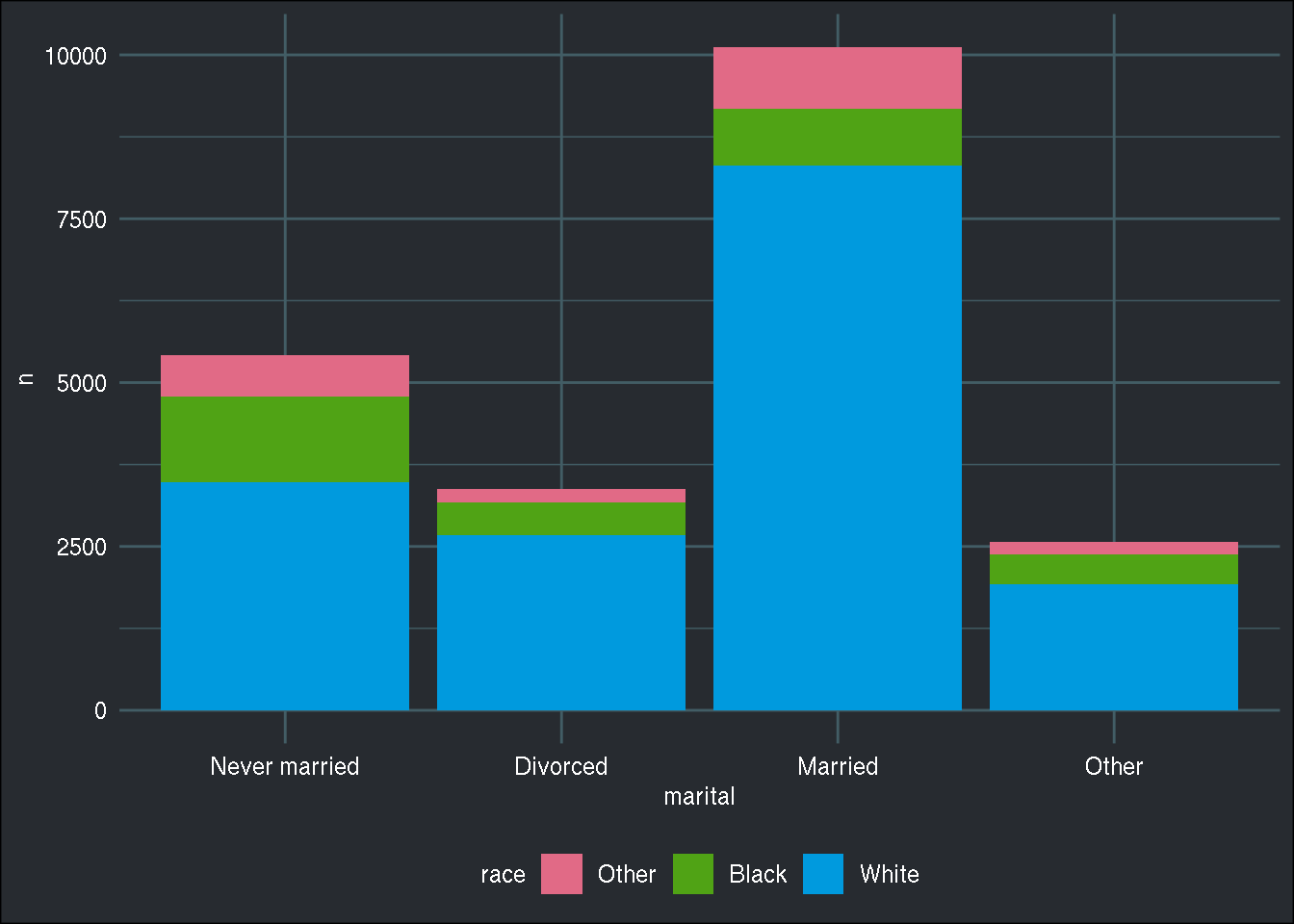Now with `position = "dodge"`:

``````ggplot(counts_marital_race) +
geom_col(aes(x = marital, y = n, fill = race), position = "dodge") +
scale_fill_discrete_qualitative(palette = "Dark 3") +
brotools::theme_blog()``````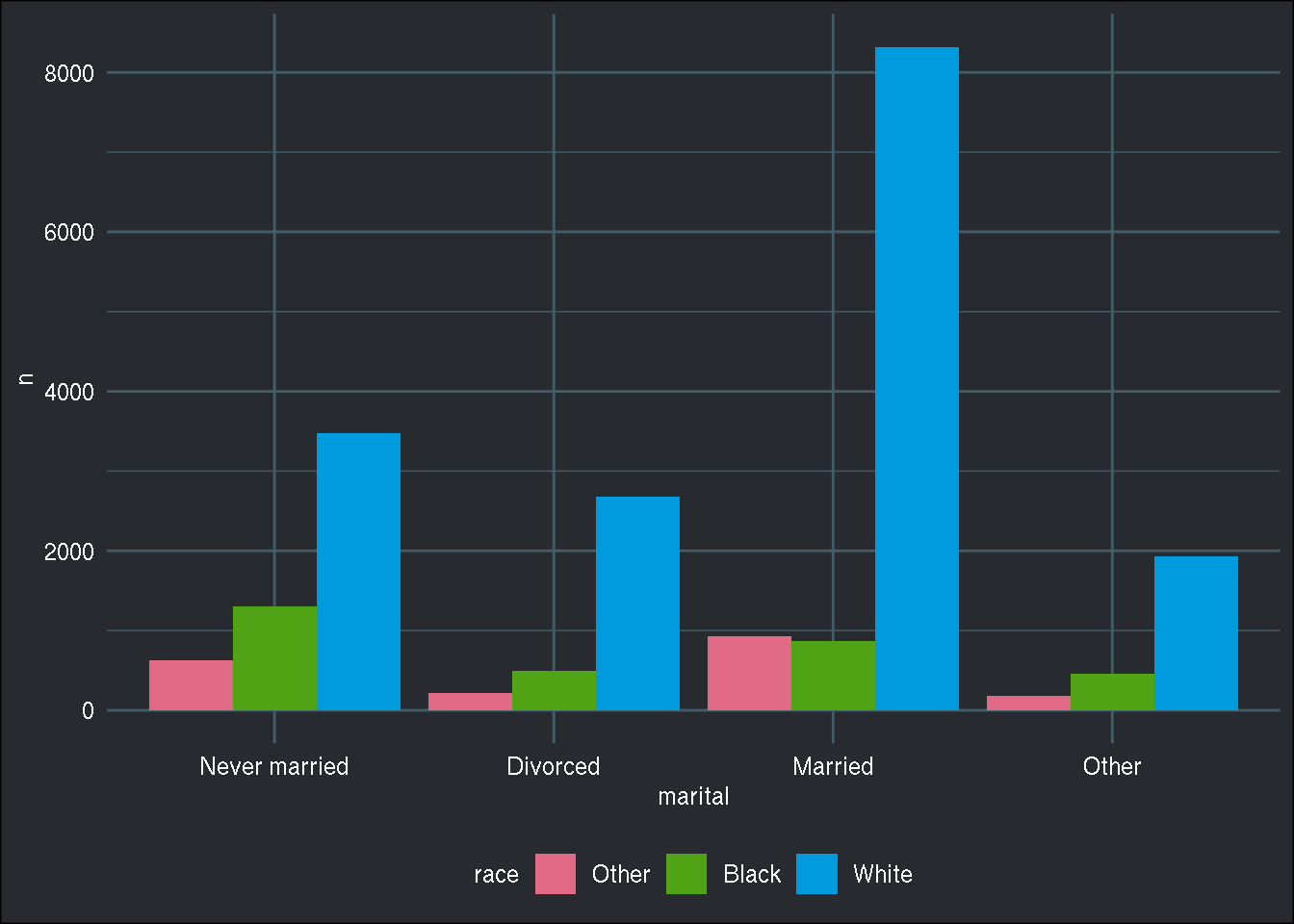Moving the legend around with `theme(legend.position = ...)`:

``````ggplot(counts_marital_race) +
geom_col(aes(x = marital, y = n, fill = race), position = "dodge") +
scale_fill_discrete_qualitative(palette = "Dark 3") +
brotools::theme_blog() +
theme(legend.position = "left")``````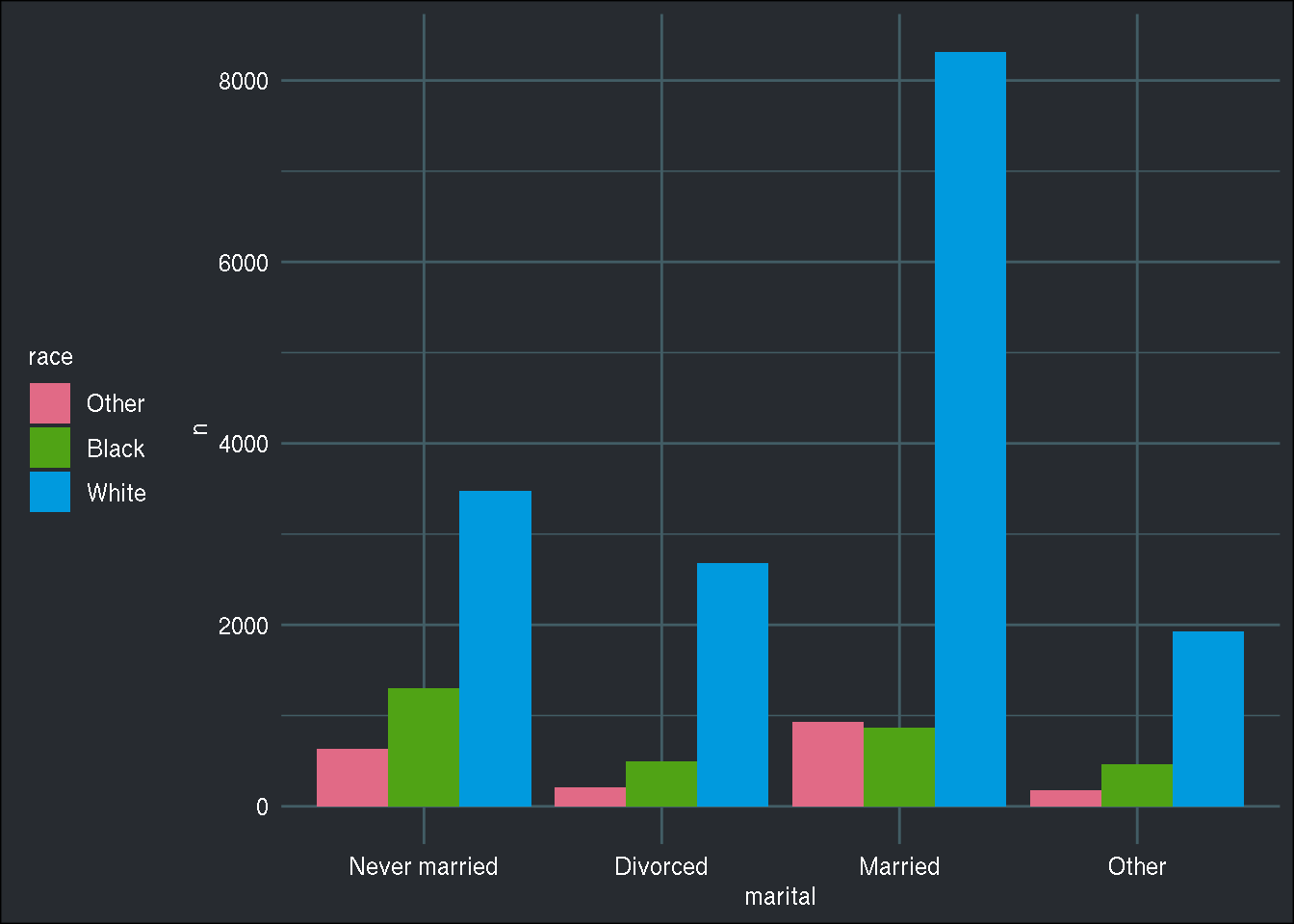Counting by year as well:

``````(
counts_marital_race_year <- gss_cat %>%
mutate(marital = fct_lump(marital, prop = 0.1)) %>%
count(year, marital, race) %>%
ungroup()
)``````
``````## # A tibble: 96 x 4
##     year marital       race      n
##  * <int> <fct>         <fct> <int>
##  1  2000 Never married Other    60
##  2  2000 Never married Black   157
##  3  2000 Never married White   495
##  4  2000 Divorced      Other    20
##  5  2000 Divorced      Black    60
##  6  2000 Divorced      White   361
##  7  2000 Married       Other    78
##  8  2000 Married       Black   121
##  9  2000 Married       White  1079
## 10  2000 Other         Other    17
## # … with 86 more rows``````

When you want to show how a variable evolves through time, area chart are handy:

``````counts_marital_race_year %>%
group_by(year, marital) %>%
summarise(n = sum(n)) %>%
ggplot() +
geom_area(aes(x = year, y = n, fill = marital)) +
scale_fill_discrete_qualitative(palette = "Dark 3") +
brotools::theme_blog() ``````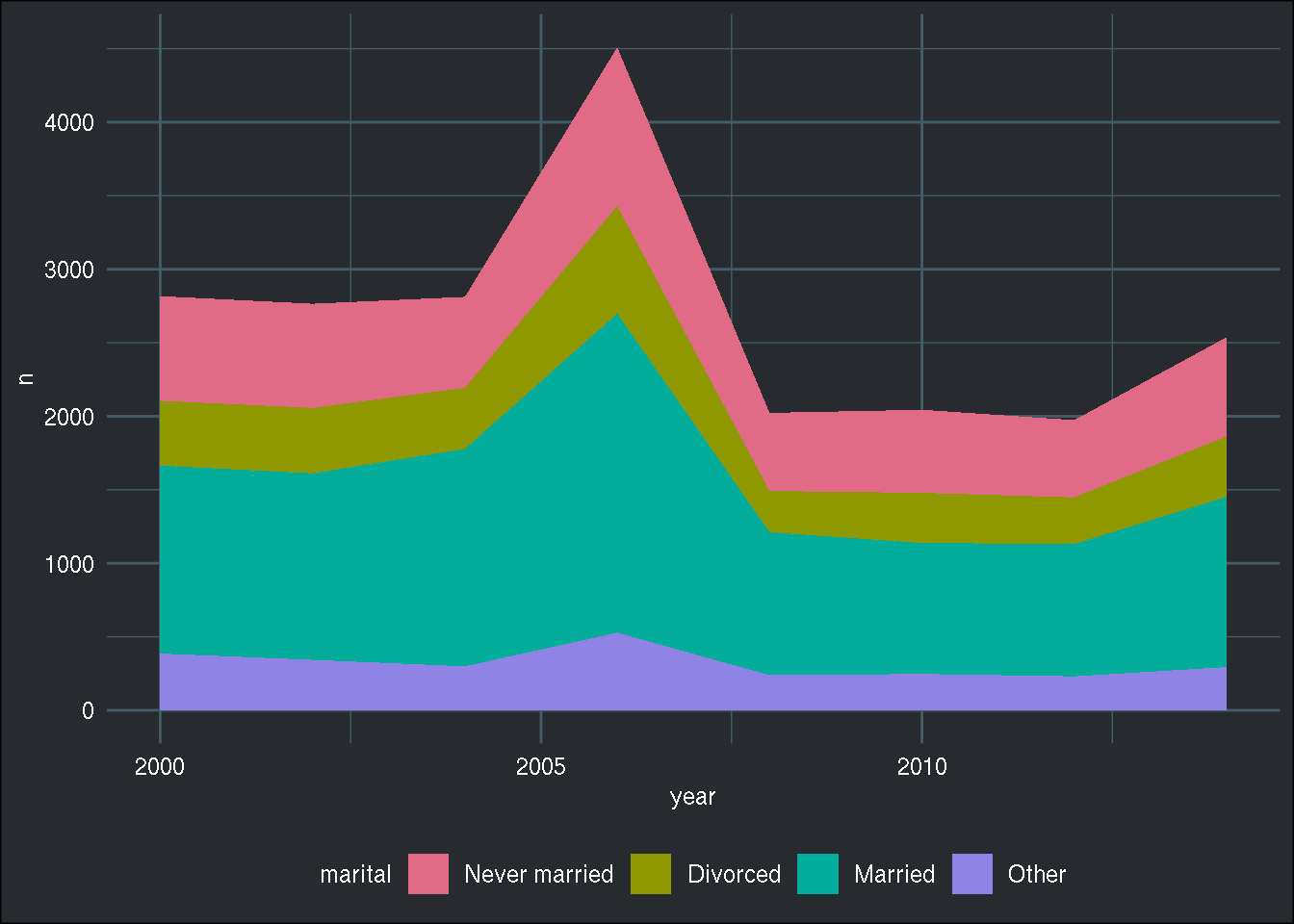Now with facets:

``````counts_marital_race_year %>%
ggplot() +
geom_area(aes(x = year, y = n, fill = marital)) +
facet_wrap(facets = vars(race), ncol = 1) +
scale_fill_discrete_qualitative(palette = "Dark 3") +
brotools::theme_blog() ``````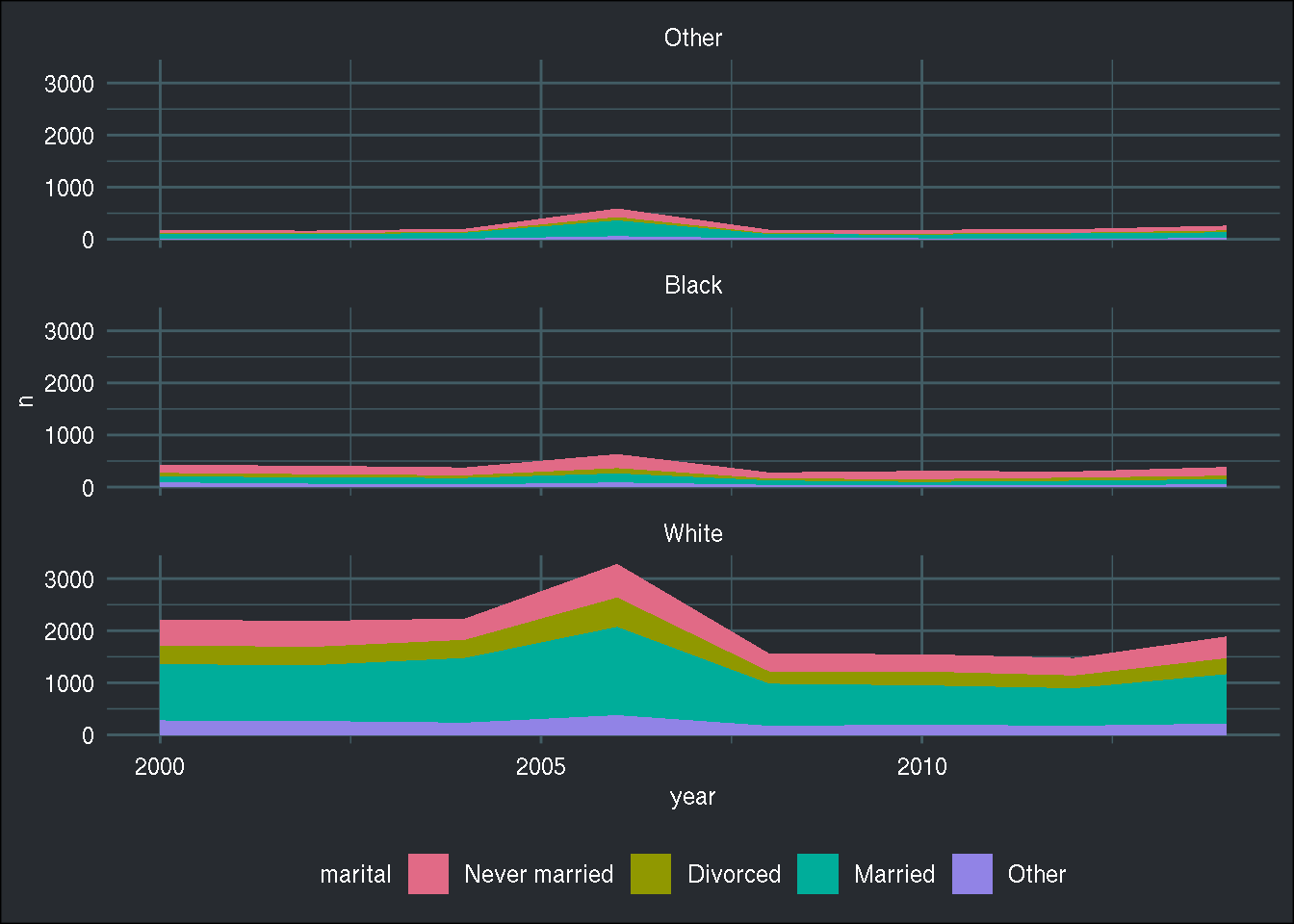But what if I want each plot to have its own y axis?

``````counts_marital_race_year %>%
ggplot() +
geom_area(aes(x = year, y = n, fill = marital)) +
facet_wrap(facets = vars(race), ncol = 1, scales = "free_y") +
scale_fill_discrete_qualitative(palette = "Dark 3") +
brotools::theme_blog() ``````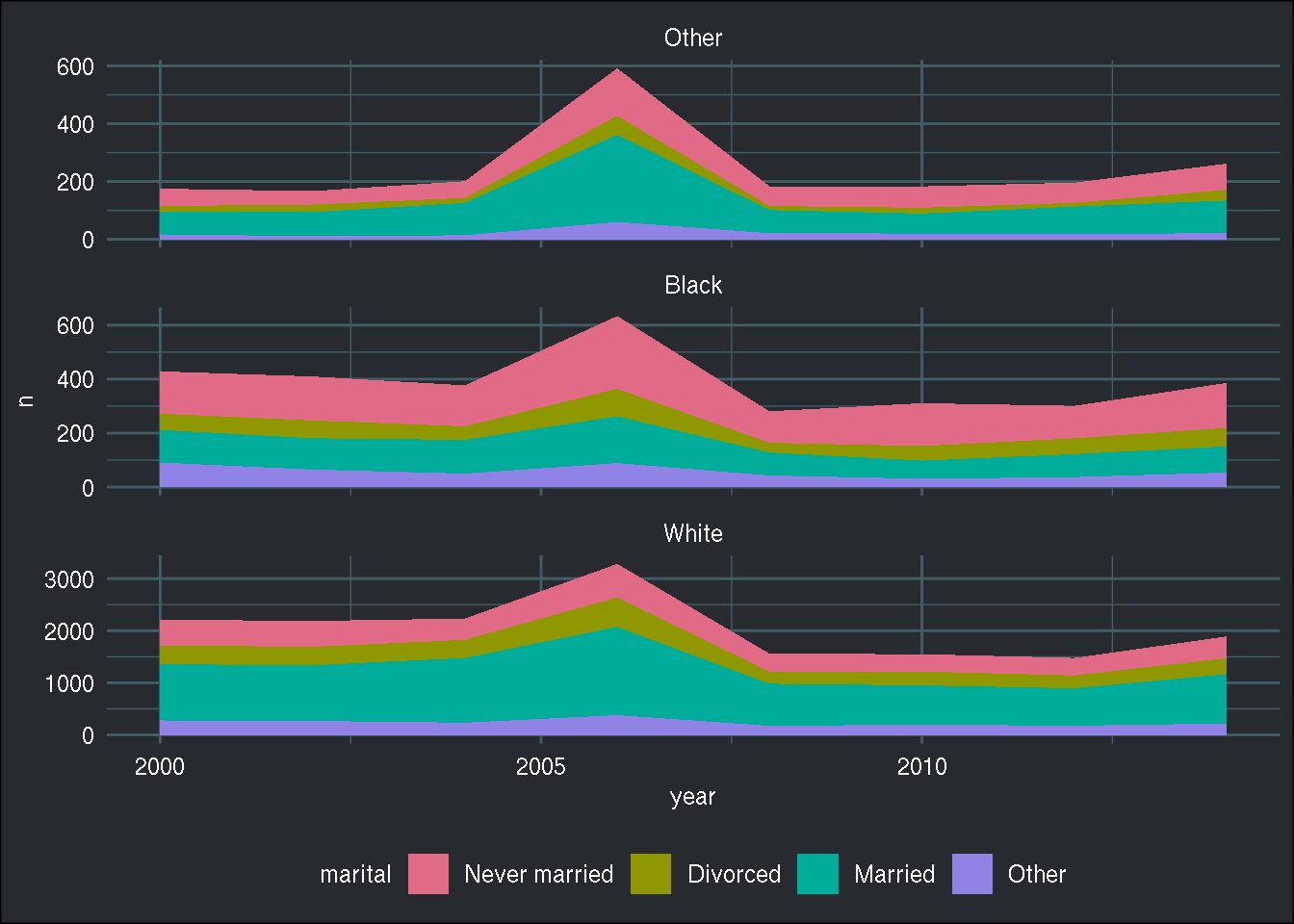Now doing an area chart but with relative frequencies:

``````counts_marital_race_year %>%
group_by(year, marital) %>%
summarise(n = sum(n)) %>%
mutate(freq = n/sum(n)) %>%
ggplot() +
geom_area(aes(x = year, y = freq, fill = marital)) +
scale_fill_discrete_qualitative(palette = "Dark 3") +
brotools::theme_blog() ``````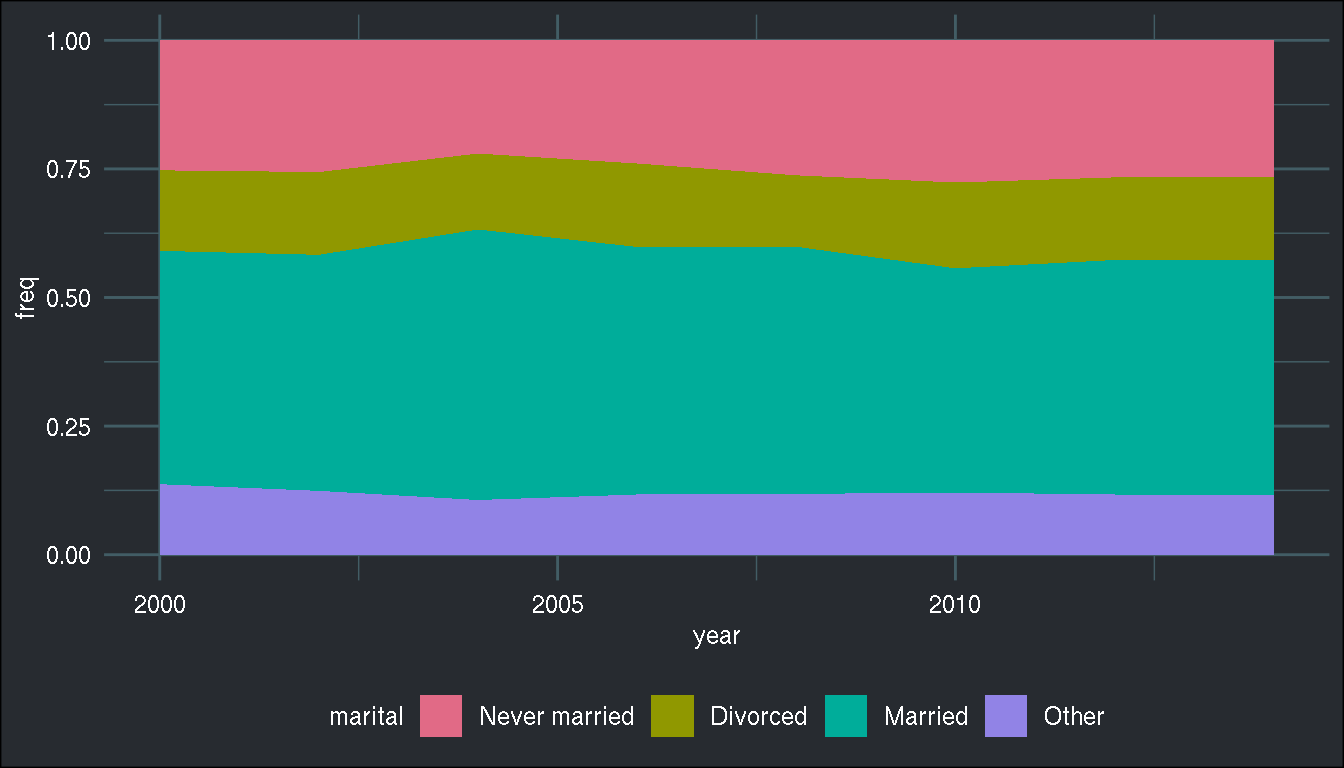With `facet_wrap()`:

``````counts_marital_race_year %>%
group_by(race, year, marital) %>%
summarise(n = sum(n)) %>%
mutate(freq = n/sum(n)) %>%
ggplot() +
geom_area(aes(x = year, y = freq, fill = marital)) +
facet_wrap(facets = vars(race), ncol = 1, scales = "free_y") +
scale_fill_discrete_qualitative(palette = "Dark 3") +
brotools::theme_blog() ``````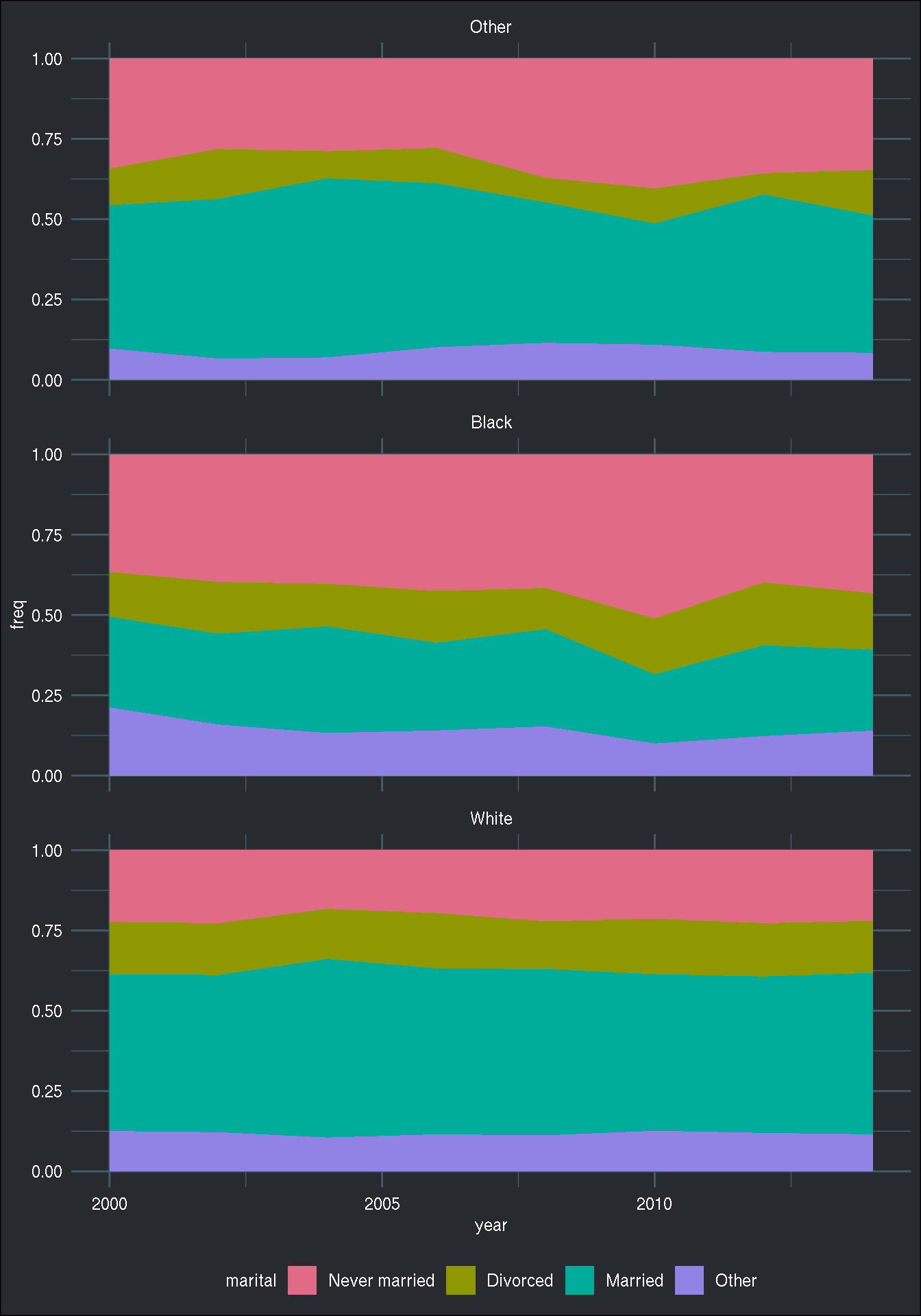Want to replace 2000 with “2000-01-01”? First need to create vector of prettier dates and positions:

``````pretty_dates <- counts_marital_race_year %>%
mutate(pretty_dates = paste0(year, "-01-01")) %>%
pull(pretty_dates) %>%
unique()

position_dates <- counts_marital_race_year %>%
pull(year) %>%
unique() %>%
sort() ``````

`scale_x_continuous()` can now use this. Using `guide = guide_axis(n.dodge = 2)` to avoid overlapping labels:

``````counts_marital_race_year %>%
group_by(race, year, marital) %>%
summarise(n = sum(n)) %>%
mutate(freq = n/sum(n)) %>%
ggplot() +
geom_area(aes(x = year, y = freq, fill = marital)) +
facet_wrap(facets = vars(race), ncol = 1, scales = "free_y") +
scale_x_continuous("Year of survey", labels = pretty_dates,
breaks = position_dates, guide = guide_axis(n.dodge = 2)) +
scale_fill_discrete_qualitative(palette = "Dark 3") +
brotools::theme_blog() ``````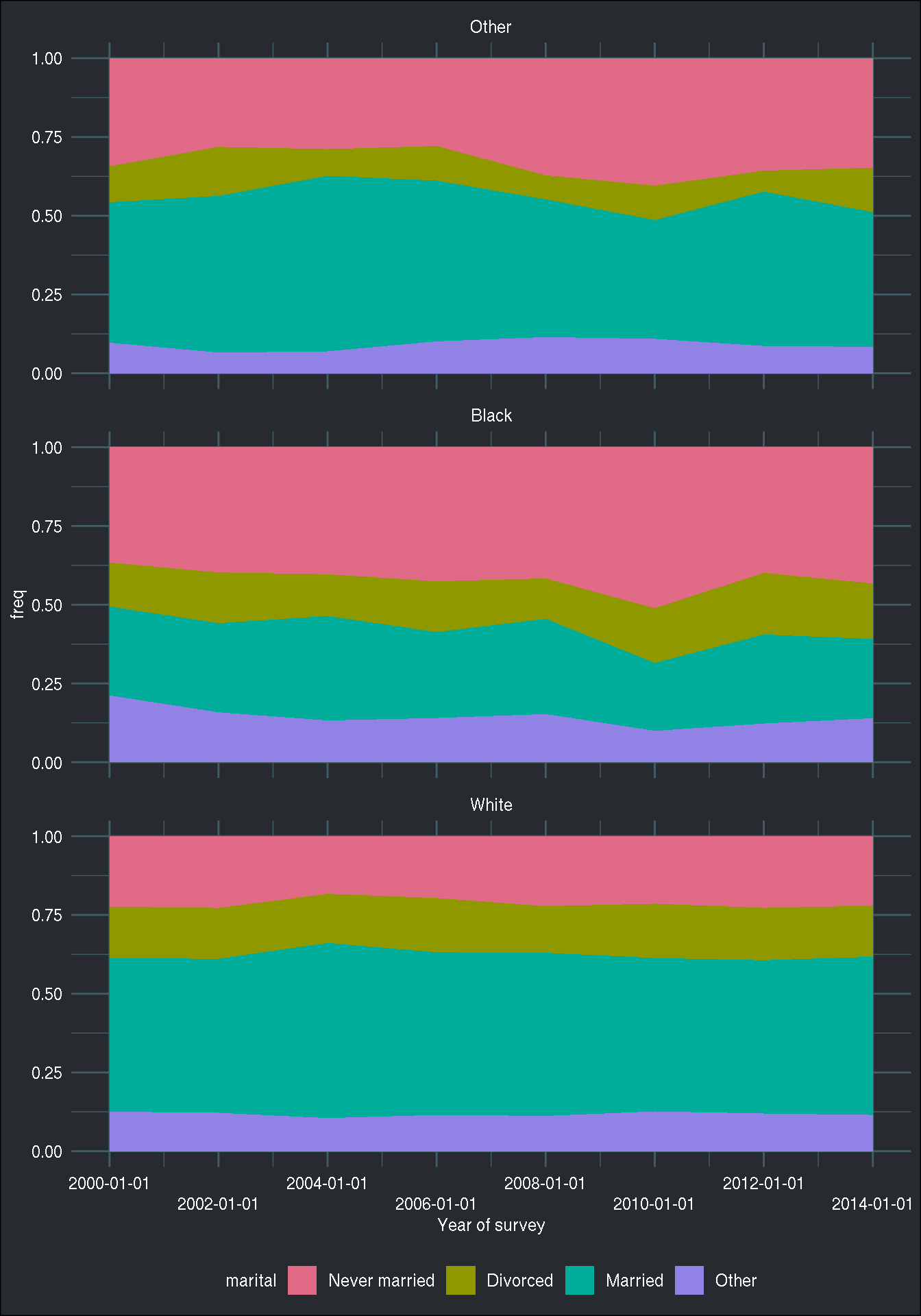Adding labels is not trivial. Here it is not working:

``````counts_marital_race_year %>%
group_by(race, year, marital) %>%
summarise(n = sum(n)) %>%
mutate(freq = n/sum(n)) %>%
ggplot() +
geom_area(aes(x = year, y = freq, fill = marital)) +
facet_wrap(facets = vars(race), ncol = 1, scales = "free_y") +
scale_x_continuous("Year of survey", labels = pretty_dates,
breaks = position_dates, guide = guide_axis(n.dodge = 2)) +
geom_label(aes(x = year, y = freq, label = round(100 * freq))) +
scale_fill_discrete_qualitative(palette = "Dark 3") +
brotools::theme_blog() ``````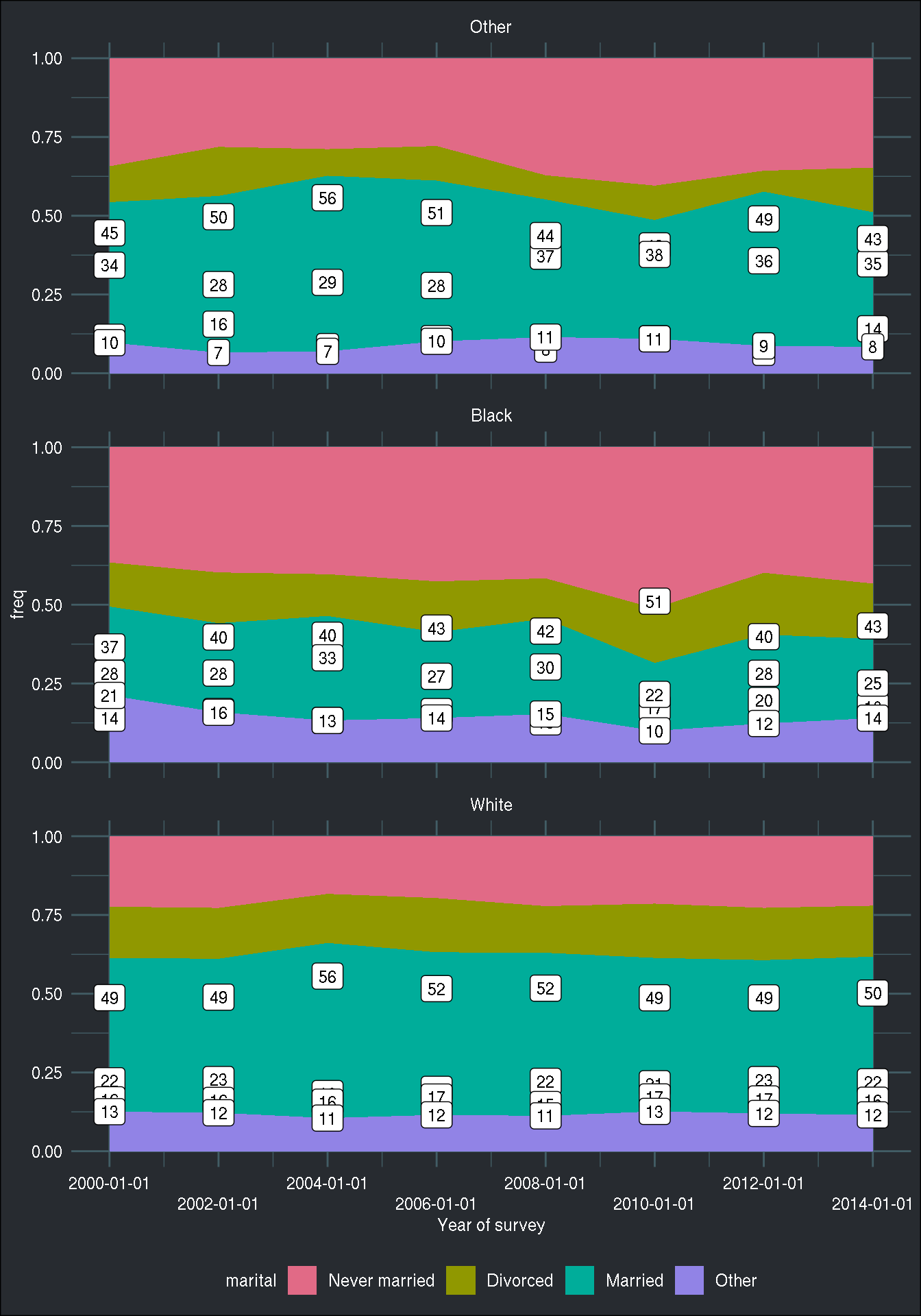Another failed attempt. I leave it here for posterity. My first idea was first to sort the grouped data set by descending frequency, and then to reorder the factor variable `marital` by descending position, which is the cumulative percentage. This would work fine, if the same factor levels would have had the same order for each of the race categories. However, this is not the case. For blacks, the most frequent category is “Never Married”. As you can see below, this trick worked well for 2 categories out of 3:

``````counts_marital_race_year %>%
group_by(race, year, marital) %>%
summarise(n = sum(n)) %>%
mutate(freq = n/sum(n)) %>%
group_by(year, race) %>%
arrange(desc(freq)) %>%
mutate(position = cumsum(freq)) %>%
mutate(marital = fct_reorder(marital, desc(position))) %>%
ggplot() +
geom_area(aes(x = year, y = freq, fill = marital)) +
facet_wrap(facets = vars(race), ncol = 1, scales = "free") +
scale_x_continuous("Year of survey", labels = pretty_dates,
breaks = position_dates, guide = guide_axis(n.dodge = 2)) +
geom_label(aes(x = year, y = position, label = round(100 * freq))) +
scale_fill_discrete_qualitative(palette = "Dark 3") +
brotools::theme_blog() ``````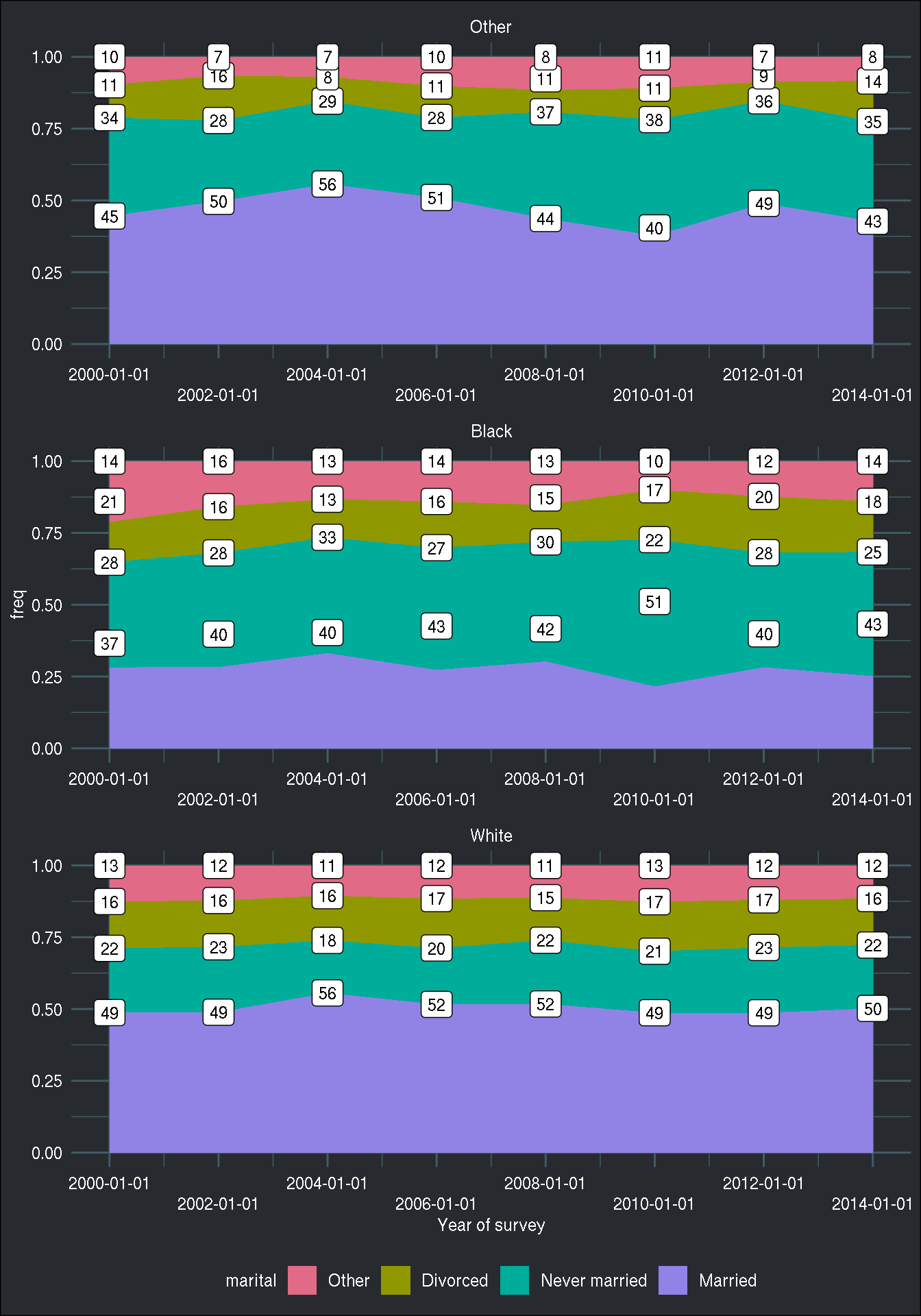So to remedy this, is not reorder too early; first, we need to reorder the factor variable by frequency. Then, we arrange the data by the now reordered `marital` variable, and then we can compute the position using the cumulative frequency.

``````counts_marital_race_year %>%
group_by(race, year, marital) %>%
summarise(n = sum(n)) %>%
mutate(freq = n/sum(n)) %>%
group_by(year, race) %>%
mutate(marital = fct_reorder(marital, freq)) %>%
arrange(desc(marital)) %>%
mutate(position = cumsum(freq)) %>%
ggplot() +
geom_area(aes(x = year, y = freq, fill = marital)) +
facet_wrap(facets = vars(race), ncol = 1, scales = "free") +
scale_x_continuous("Year of survey", labels = pretty_dates,
breaks = position_dates, guide = guide_axis(n.dodge = 2)) +
geom_label(aes(x = year, y = position, label = round(100 * freq))) +
scale_fill_discrete_qualitative(palette = "Dark 3") +
brotools::theme_blog() ``````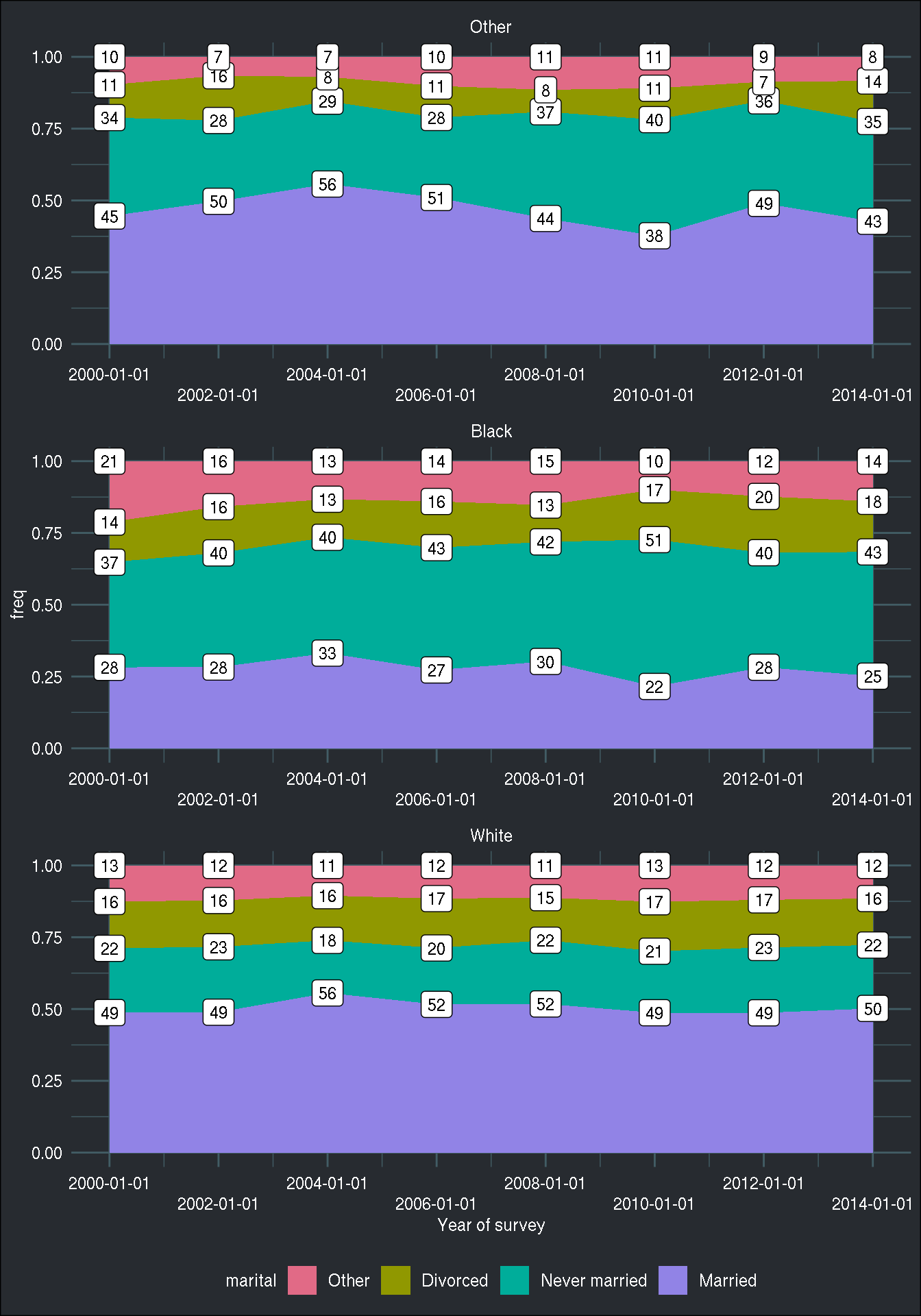We can place the labels a bit better (in the middle of their respective areas), like so:

``````counts_marital_race_year %>%
group_by(race, year, marital) %>%
summarise(n = sum(n)) %>%
mutate(freq = n/sum(n)) %>%
group_by(year, race) %>%
mutate(marital = fct_reorder(marital, freq)) %>%
arrange(desc(marital)) %>%
mutate(position = cumsum(freq)) %>% mutate(prev_pos = lag(position, default = 0)) %>%
mutate(position = (position + prev_pos)/2) %>%
ggplot() +
geom_area(aes(x = year, y = freq, fill = marital)) +
facet_wrap(facets = vars(race), ncol = 1, scales = "free") +
scale_x_continuous("Year of survey", labels = pretty_dates,
breaks = position_dates, guide = guide_axis(n.dodge = 2)) +
geom_label(aes(x = year, y = position, label = round(100 * freq))) +
scale_fill_discrete_qualitative(palette = "Dark 3") +
brotools::theme_blog() ``````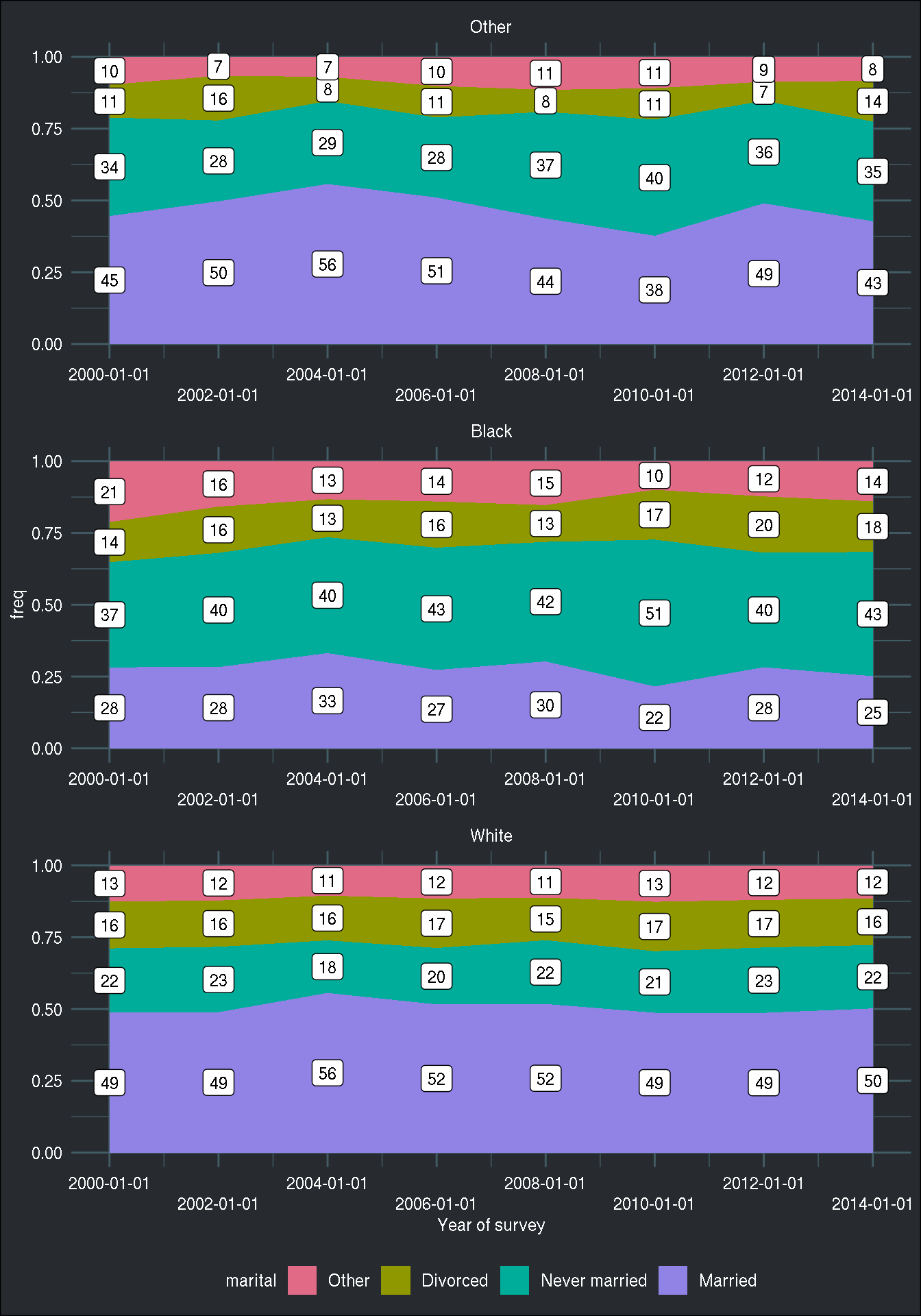Now let’s focus on the variable `tvhours`. We want to show the total watched hours, but also the total across all the categories of `race` and `marital` in a faceted bar plot:

``````(
total_tv <- gss_cat %>%
group_by(year, race, marital) %>%
summarise(total_tv = sum(tvhours, na.rm = TRUE))
)``````
``````## # A tibble: 127 x 4
## # Groups:   year, race 
##     year race  marital       total_tv
##    <int> <fct> <fct>            <int>
##  1  2000 Other No answer            2
##  2  2000 Other Never married      103
##  3  2000 Other Separated           16
##  4  2000 Other Divorced            17
##  5  2000 Other Widowed             24
##  6  2000 Other Married            122
##  7  2000 Black Never married      452
##  8  2000 Black Separated          135
##  9  2000 Black Divorced           156
## 10  2000 Black Widowed            183
## # … with 117 more rows``````

This tibble has the total watched hours by year, race and marital status variables. How to add the total by year and race categories? For this, by are first going to use the `group_split()`:

``````total_tv_split <- total_tv %>%
select(race, year, marital, total_tv) %>%
mutate(year = as.character(year)) %>%
group_split(year, race)``````
``````## Warning: ... is ignored in group_split(<grouped_df>), please use
## group_by(..., .add = TRUE) %>% group_split()``````

I have to re-order the columns with `select()`, because when using `janitor::adorn_totals()`, which I will be using below to add totals, the first column must be a character column (it serves as an identifier column).

This creates a list with 3 races times 6 years, so 24 elements. Each element of the list is a tibble with each unique combination of year and race:

``length(total_tv_split)``
``##  24``
``total_tv_split[1:2]``
``````## <list_of<
##   tbl_df<
##     race    : factor<f4a07>
##     year    : character
##     marital : factor<82ceb>
##     total_tv: integer
##   >
## >>
## []
## # A tibble: 6 x 4
##   race  year  marital       total_tv
##   <fct> <chr> <fct>            <int>
## 1 Other 2000  No answer            2
## 2 Other 2000  Never married      103
## 3 Other 2000  Separated           16
## 4 Other 2000  Divorced            17
## 5 Other 2000  Widowed             24
## 6 Other 2000  Married            122
##
## []
## # A tibble: 5 x 4
##   race  year  marital       total_tv
##   <fct> <chr> <fct>            <int>
## 1 Black 2000  Never married      452
## 2 Black 2000  Separated          135
## 3 Black 2000  Divorced           156
## 4 Black 2000  Widowed            183
## 5 Black 2000  Married            320``````

Why do this? To use `janitor::adorn_totals()`, which adds row-wise totals to a data frame, or to each data frame if a list of data frames gets passed to it. I need to still transform the data a little bit. After using `adorn_totals()`, I bind my list of data frames together, and then fill down the year column (when using `adorn_totals()`, character columns like `year` are filled with `"-"`, but I chose to fill it with `NA_character_`). I then replace the NA value from the marital column with the string `"Total"` and then reorder the `marital` column by value of `total_tv`:

``````total_tv_split <- total_tv_split %>%
map(as.data.frame) %>%
bind_rows() %>%
fill(year, .direction = "down") %>%
mutate(marital = ifelse(is.na(marital), "Total", marital)) %>%
mutate(marital = fct_reorder(marital, total_tv))``````

I can finally create my plot. Because I have added “Total” as a level in the `marital` column, it now appears seamlessly in the plot:

``````ggplot(total_tv_split) +
geom_col(aes(x = marital, y = total_tv, fill = race)) +
facet_wrap(facets = vars(year), nrow = 2) +
scale_fill_discrete_qualitative(palette = "Dark 3") +
scale_x_discrete(guide = guide_axis(n.dodge = 3)) +
brotools::theme_blog() ``````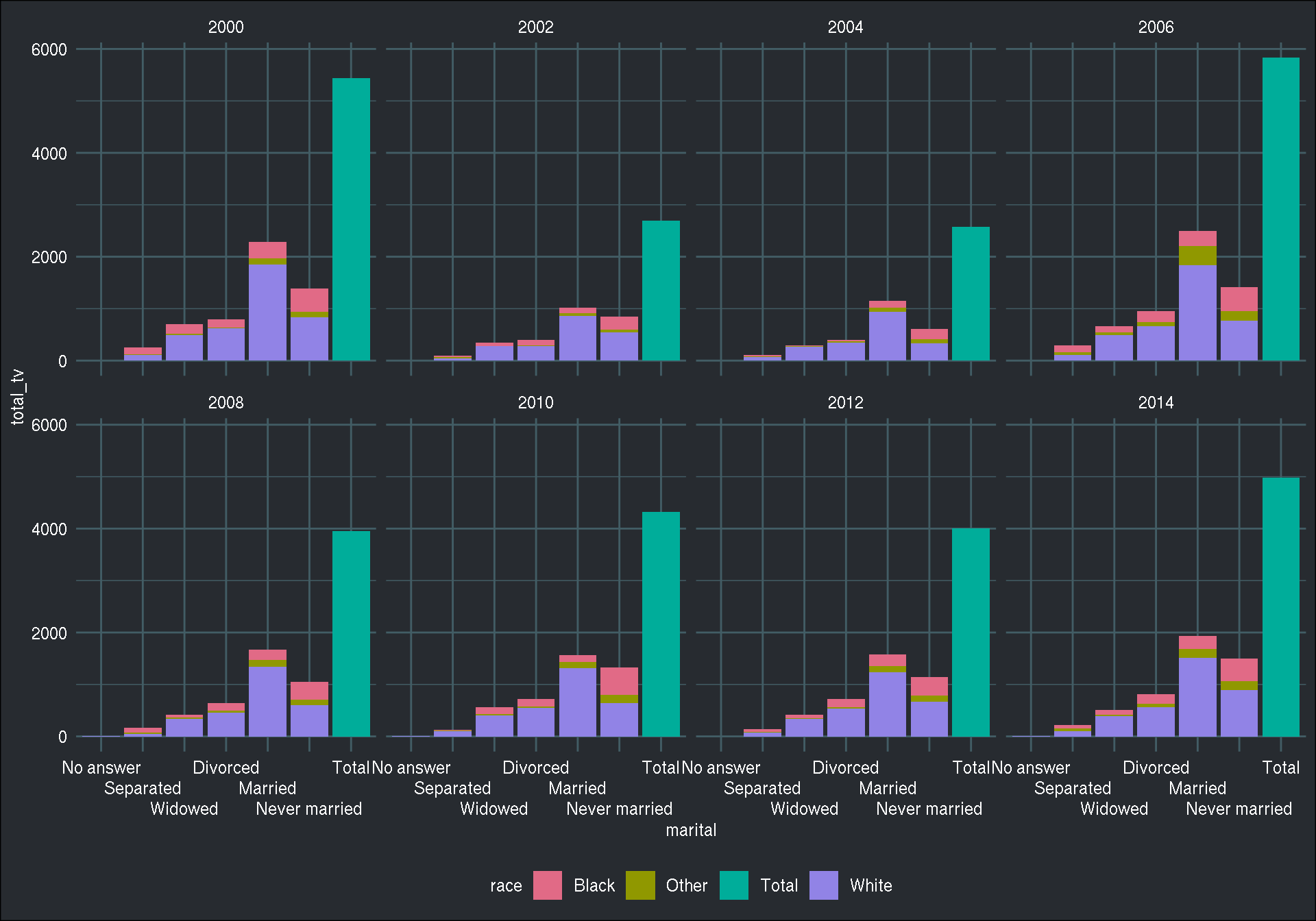To finish this list of recipes, let’s do a pyramid plot now (inspiration from here:

``````data_pyramid <- gss_cat %>%
filter(year == "2000", marital %in% c("Married", "Never married")) %>%
group_by(race, marital, rincome) %>%
summarise(total_tv = sum(tvhours, na.rm = TRUE))

ggplot(data_pyramid, aes(x = rincome, y = total_tv, fill = marital)) +
geom_col(data = filter(data_pyramid, marital == "Married")) +
geom_col(data = filter(data_pyramid, marital == "Never married"), aes(y = total_tv * (-1))) +
facet_wrap(facets = vars(race), nrow = 1, scales = "free_x") +
coord_flip() +
scale_fill_discrete_qualitative(palette = "Dark 3") +
brotools::theme_blog() ``````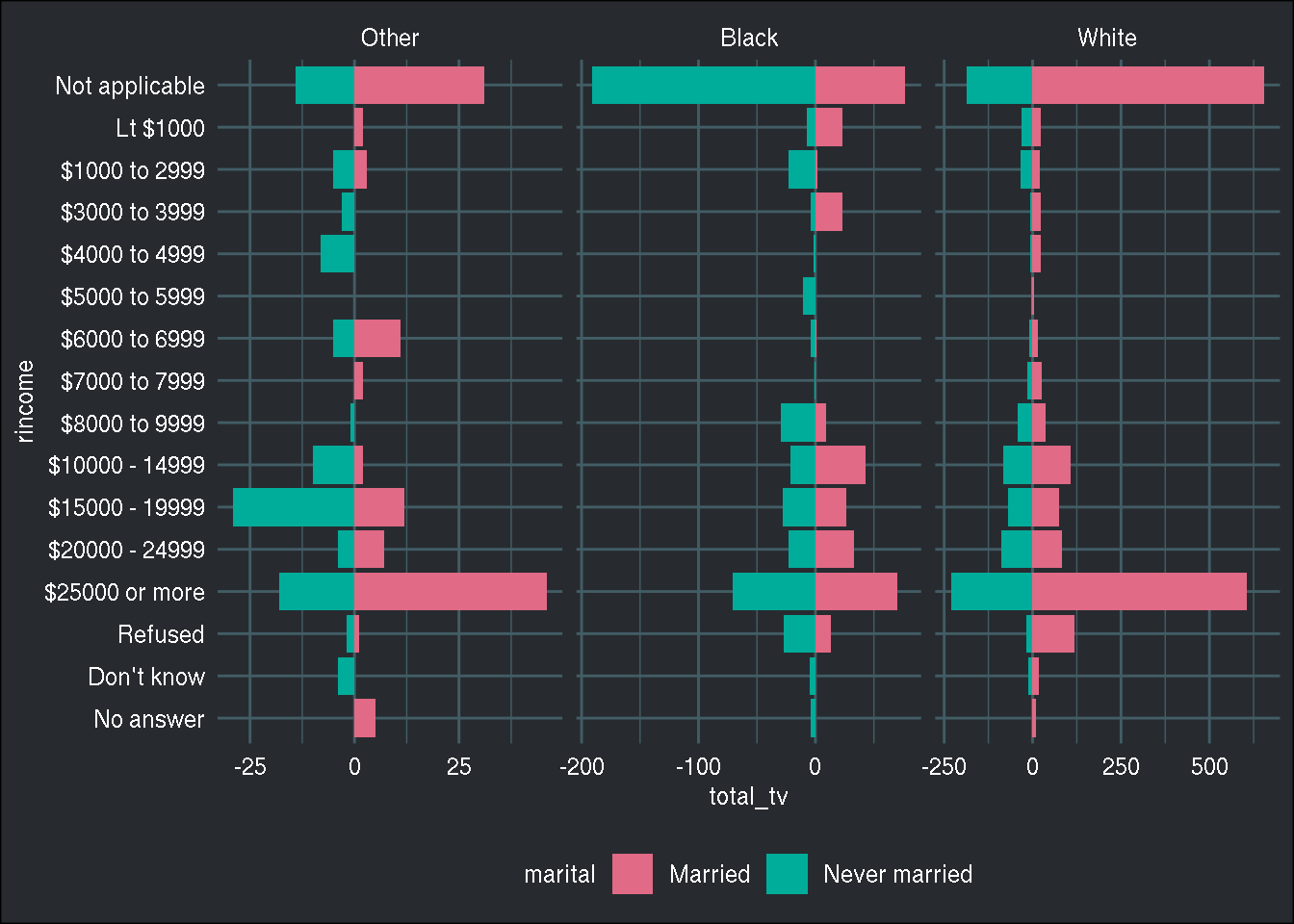Happy Easter!

Hope you enjoyed! If you found this blog post useful, you might want to follow me on twitter for blog post updates and buy me an espresso or paypal.me, or buy my ebook on Leanpub.